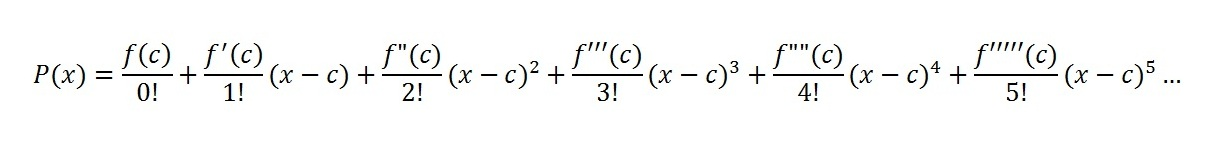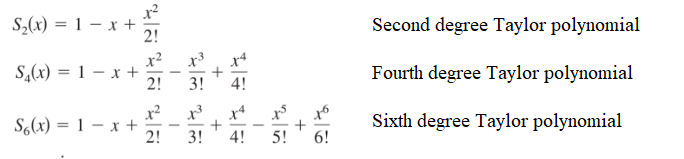# nth Degree Taylor PolynomialMany functions can be approximated with an nth degree Taylor polynomial.An nth degree Taylor polynomial (named after the 17th century English mathematician Brook Taylor) is a way to approximate a function with a partial sum— a series of additions and multiplications. The “nth” refers to the degree of the polynomial you’re using to approximate the function.

Many transcendental functions (e.g. logarithmic functions or trigonometric functions) can be expressed as these approximations; When you plug in cos 35° to a calculator, it doesn’t use a table to find that value: it uses a polynomial approximation.

## Example of nth degree Taylor Polynomial

The function f(x) = e-x can be represented by an nth degree Taylor polynomial. The higher the “n”(degree), the better the approximation.If it were possible to write an infinite number of degrees, you would have an exact match to your function. However, the goal here (like in many areas of calculus) isn’t to be “exact”, but to have an approximation that’s “good enough.” As you can’t write out an infinite number of sums, you choose a workable part (perhaps 10 or 20): this small part is called a partial sum.

The following graph shows a tenth degree polynomial does a fairly good job at approximating the function (better than the sixth degree polynomial). Depending on what part of the function you’re working with, this may be good enough.## How Many Degrees is Good Enough?

Even the tenth degree (in blue) doesn’t do well at approximating the tail on the right of the above graph, so if you’re evaluating a function there you will want to go even higher with the number of degrees. How high should you go? How many terms is good enough? That can be answered with Taylor’s inequality, which states that (Lahodny, n.d.):

If |f(n + 1)(x) | ≤ M for |x – a| < R, then the remainder Rn(x) of the Taylor series satisfies## What’s the Difference Between a Taylor Polynomial and a Taylor Series?

A Taylor Series is the entire infinite sum of additions and multiplications, which can be represented in summation notation byWhen you take a part of this series and create a smaller number of partial sums (like the ones I used above to graph approximations for e-x, those sums are called nth degree Taylor polynomials (or just “Taylor Polynomials” for short).

## References

Lahodny, G. (2019). Section 10.9: Applications of Taylor Polynomials. Retrieved July 21, 2020 from: https://www.math.tamu.edu/~glahodny/Math152/Section%2010.9.pdf
Schwartz, S. (2017). AP® Calculus AB & BC All Access Book. Research & Education Association.
Graph: Demos.com

CITE THIS AS:
Stephanie Glen. "nth Degree Taylor Polynomial" From StatisticsHowTo.com: Elementary Statistics for the rest of us! https://www.statisticshowto.com/nth-degree-taylor-polynomial/